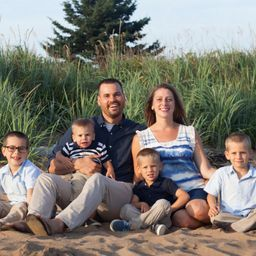# Joshua E.

Other Schools

## Biography

I obtained a BSc in Mathematics and Chemistry, then a BEd in teaching mathematics, and I have been teaching it for the last 13 years. I teach mostly advanced Algebra and Calculus courses, though have also taught upper level Physics. I love math, and love to share my love of math with others. My LinkedIn porfile is at https://www.linkedin.com/in/josh-eastwood-108a9a143/

BS Mathematics
Other Schools

## Topics Covered

Functions
Trigonometry
Equations and Inequalities
Introduction to Trigonometry
Introduction to Conic Sections
Introduction to Conic Section
Polar Coordinates
Partial Derivatives
Functions of Several Variables
Rational Functions
Sequences
Series
Introduction to Sequences and Series
Functions
Exponents and Polynomials
Polynomials
Matrices
Systems of Equations and Inequalities
Introduction to Matrices
Exponential and Logarithmic Functions
An Introduction to Geometry
Limits
Differentiation
Continuous Functions
Graphs and Statistics
Algebra Topics That are Reviewed at the Start of the Semester
Complex Numbers
Introduction to Algebra
Introduction to Sequences and Series
Derivatives
Applications of the Derivative
Differential Equations
Integration
Integration Techniques
Introduction to Combinatorics and Probability
Introduction to Combinatorics and Probability
1 2 3 4 5 ... 392